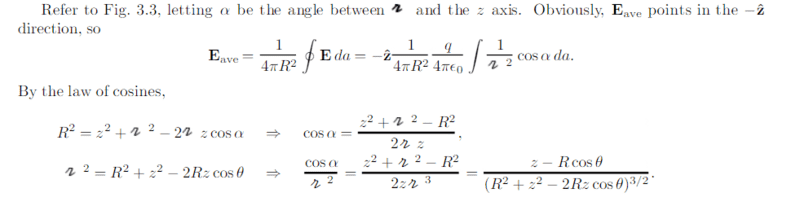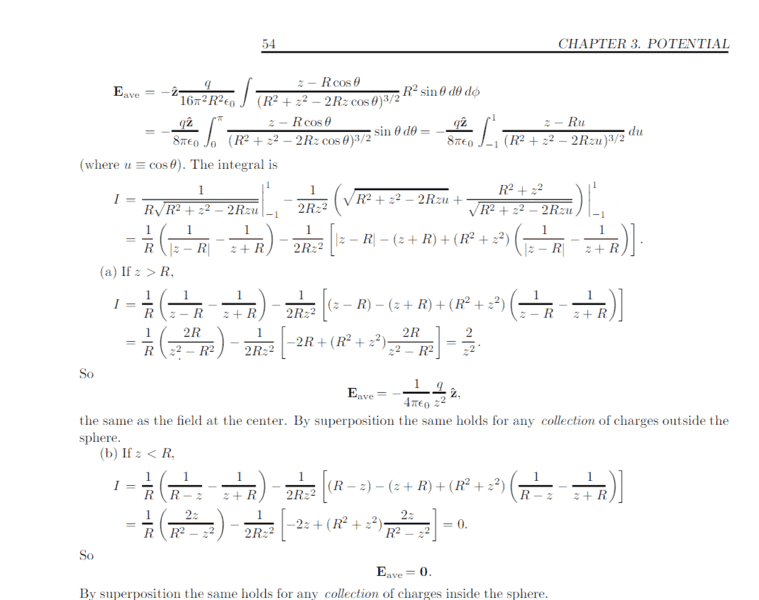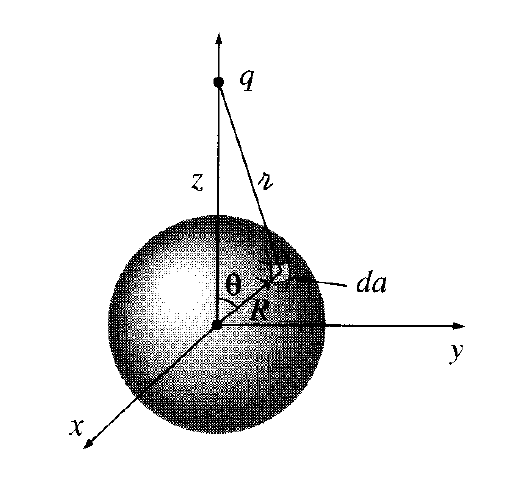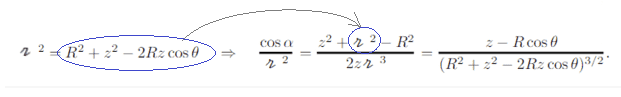# Average electric field over a spherical surface

## Homework Statement

I was working out problem 4, chapter 3 of Introduction to Electrodynamics by Griffiths:

a) Show that the average electric field over a spherical surface, due to charges outside the sphere, is the same as the field at the centre.

b) What is the average due to charges inside the sphere?

## The Attempt at a Solution

I know the solution, but there are a few aspects I do not see:Here you have the image Griffiths makes reference at:Why ##z^2 + r^2 - R^2 = z - Rcos \theta## (law of cosines developement)?

#### Attachments

TSny
Homework Helper
Gold Member
Why ##z^2 + r^2 - R^2 = z - Rcos \theta## (law of cosines developement)?
This equality is not correct. Instead, note the following substitution#### Attachments

•JD_PM